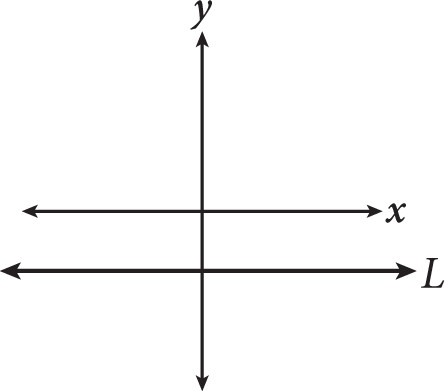# SAT Math Multiple Choice Question 274: Answer and Explanation

### Test Information

Question: 274

4.Line L shown in the graph could be the graph of which equation?

• A. x + y = -2
• B. x + y = 0
• C. x + y - 2 = x
• D. x + y + 2 = x